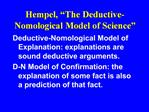DownloadDownload PresentationHempel, The Deductive-Nomological Model of Science

# Hempel, The Deductive-Nomological Model of Science

Download Presentation## Hempel, The Deductive-Nomological Model of Science

- - - - - - - - - - - - - - - - - - - - - - - - - - - E N D - - - - - - - - - - - - - - - - - - - - - - - - - - -
##### Presentation Transcript

1. Hempel, �The Deductive-Nomological Model of Science� Deductive-Nomological Model of Explanation: explanations are sound deductive arguments. D-N Model of Confirmation: the explanation of some fact is also a prediction of that fact.

2. D-N Model of Explanation explanandum sentence: sentence describing the event or phenomenon to be explained; explanans sentences: contain the explanatory information; In a good explanation, the explanans deductively implies the explanandum.

3. Explanans sentences The explanans consists of two kinds of sentences: 1. General laws that express uniform empirical connections. 2. Descriptions of facts.

4. Schematic for D-N Explanation L1,...,Ln (General laws) C1,...,Cm (Descriptions of facts) E (Explanandum event)

5. Summary on Explanation Hempel�s examples are designed to show that the D-N model accounts for how both particular facts and uniformities in nature can be explained by science. Namely, by using a deductive argument in which the thing to be explained, the explanandum, is the conclusion.

6. D-N Model of Confirmation The explanation of some fact is also a prediction of that fact. When a prediction is born out by observation, that is some confirming evidence for the theory.

7. Problem for D-N Confirmation The evidence for a theory is minimal and mainly negative, based on the following modus tollens argument: P1. If (L1,...,Ln and C1,...,Cm) then E. P2. Not E. C. Therefore, not (L1,...,Ln and C1,...,Cm)

8. Modus Tollens Modus Tollens is a valid deductive argument form: If p then q. Not q. Therefore not p.

9. Example of Modus Tollens If the body theory of personal identity is correct, then one is not the same person whenever one loses body parts. But losing one�s hand does not make one a different person. Therefore the body theory of personal identity is not correct.

10. Structure and Purpose of Scientific Theories explain a set of uniformities as a manifestation of some underlying processes and entities. specify clearly and precisely the basic entities and processes that occur in nature examples: Newtonian physics; kinetic theory of gases

11. Theories, continued This clarity and precision allows deduction of regularities and hence tests of the theory. Thus the clarity and precision of scientific theories sets science apart from unscientific theories.

12. Derivation of Predictions Theory must specify both internal principles (which characterize basic entities, processes, and laws of theory) and bridge principles. The bridge principles tie the theoretical terms to the pre-theoretical or everyday terms. Prediction is derived by deduction from theory and initial conditions.

13. Virtues of Theories Theories broaden and deepen understanding of nature in three ways: 1. offer a systematic, unified account of diverse phenomena 2. show how previous theories hold approximately 3. predict and explain new(ly discovered) phenomena

14. Problems with Hempel�s Picture of Science 1. Most predictions/explanations in science are not deductive This led Hempel to introduce the Inductive-Statistical model: L1,...,Ln C1,...,Cm implies with high probablility: E

15. Problems, continued 2. However, as Popper argues, induction is a flimsy method of confirmation of theories or laws 3. Laws are astonishingly difficult to characterize